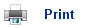# Energy changes in solutions

## Lattice Enthalpy

• The lattice enthalpy is defined as the energy given out when one mole of a solid is formed by the coming together of separate ions (gaseous).
• When ions are separated from each other, they can be thought of as being in the gaseous state; when separate ions come together to form a lattice, they are in the solid state.
• So, the lattice enthalpy can be defined as the enthalpy change involved in processes such as: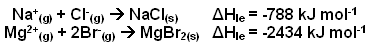• Forming a lattice from gaseous ions is always an exothermic process, and soHle is always negative.
• In order for an ionic substance to dissolve in water, the ions in the lattice must be separated; this requires energy to overcome the electrical attraction between the ions.
• The strength of the ionic attractions in the lattice is measured using the lattice energy.
• The lattice energy must be supplied in order to break down a lattice and enable the ionic substance to dissolve in water.
• This energy tends to stop substances dissolving- unless the energy is paid back in later.
• The table below shows some lattice enthalpy values for some simple ionic compounds: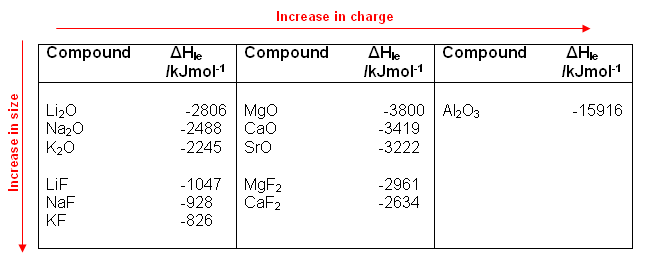• As illustrated in the table above, lattice enthalpies tend to become more negative (i.e. more energy is given out) when:
• The ionic charge increases.
• This is because the ions with a larger charge attract one another more strongly, and the smaller the ions are, the closer they can get to each other.
• Substances with large lattice enthalpies, e.g. Al2O3, tend to be insoluble.

## Hydration and Solvation

• Even though energy is needed to break up the lattice, many ionic substances do dissolve.
• Therefore, something else must happen to supply that energy.
• Water molecules possess a dipole and are attracted to the ions on the surface of the lattice when an ionic compound is added to water.
• The water molecules pull the ions from the surface of the compound; the ions in the solution become hydrated; they have water molecules bound to them: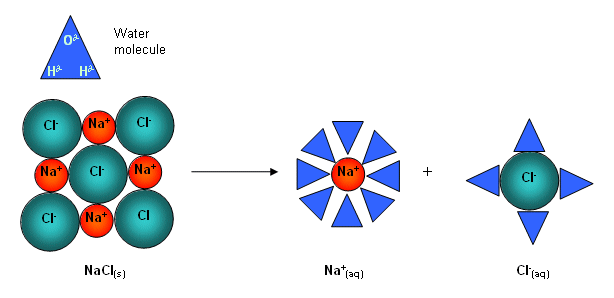• The smaller the ion and the higher its charge, the more water molecules it attracts.
• The strength of the attractions between the ions and the water molecules is measured by the enthalpy of hydration (Hhyd).
• The enthalpy change of hydration is defined as the enthalpy change for the production of a solution of ions from one mole of gaseous ions.
• For example: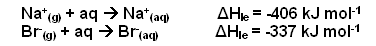• Enthalpies of hydration depend upon the concentration of the solution produced.
• Values quoted refer to an infinitely dilute solution.
• Enthalpies of hydration are always negative (exothermic).
• Once again, the most exothermic values occur when the ionic radii decreases and the ionic charge increases.
• Molecules of some other solvents, such as ethanol, are also polar and can bind to ions. When dealing with solvents other than water, the enthalpy change is referred to as the enthalpy of solvation (Hsolv).

## Enthalpy change of solution

• The hydration of ions favours dissolving and helps to supply the energy needed to separate the ions from a lattice.
• The difference between the enthalpies of hydration of ions and the lattice enthalpy gives the enthalpy change of solution (Hsolution).
• This can be measured experimentally; it is the enthalpy change when one mole of a solute dissolves to form an infinitely dilute solution.
• The process of dissolving can be broken down into two steps:
1. The solid ionic compound is split up into a gas of ions (requires the energy equal to the lattice energy to be supplied).
2. The ions dissolve in solution and become hydrated; the energy equal to the hydration energies of the two ions is given out.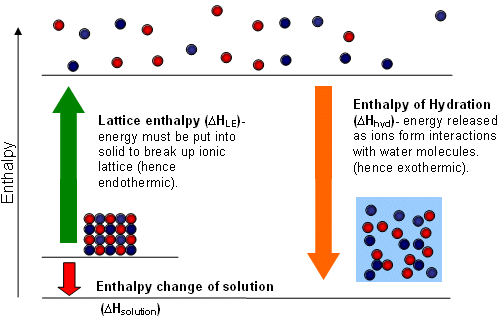• Therefore, the enthalpy of solution is equal to:H(solution) =Hhyd(cation) +Hhyd(anion) + -HLE

• The following enthalpy level diagram represents a solute for whichHsolution is slightly negative: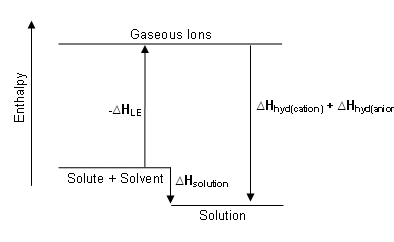• The following enthalpy level diagram represents a solute for whichHsolution is slightly positive:• The value ofHsolution depends upon the difference between the enthalpy of hydration of the individual ions and the lattice enthalpy of the compound.
• Even if the value ofHsolution is positive, the substance may still dissolve; this is related to the change in entropy of the system that occurs.
•HLE andHHyd values are often very large and are dependent on the size and charge of the ions involved.
• As a result, the values ofHsolution are often small by comparison, and can be either positive or negative.
• But even knowing the value forHsolution will not tell us whether an ionic compound will dissolve or not, as entropy also needs to be taken into account when making predictions.

## Entropy and Dissolving

• The entropy of a system increases when a solute dissolves, as it becomes more disordered as the ions spread out through the solution.
• An increase in entropy favours dissolving- even if a small amount of energy is needed.
• So substances with a small positive enthalpy change of solution are still able to dissolve, provided there is a sufficient increase in entropy.
• This can be used to explain why salt (NaCl) dissolves, but calcium carbonate (chalk) does not.
• When salt dissolves there is a large increase in entropy, which counteracts the small positive enthalpy change of solution value that it has.
• Calcium carbonate, on the other hand, has a small negative enthalpy change of solution; however a decrease in entropy results from it dissolving and so it is insoluble in water.
• The decrease in entropy occurs because the solvent, water, is becoming more ordered as the water molecules cluster around the doubly charged ions.

## Solubility of Group 2 compounds

• The enthalpy change of solution can also be used to explain the trends in the solubility of group two compounds.
• The solubility or the group 2 hydroxides increases down the group, whereas the solubility of group 2 carbonates decreases down the group.

## Useful books for revision

Revise A2 Chemistry for Salters (OCR A Level Chemistry B)Salters (OCR) Revise A2 ChemistryHome